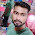### Program 280: Print Sum of Even Numbers in Array using Recursion

Program 280: Print Sum of Even Numbers in Array using Recursion

```#include<stdio.h>
void SumOfEven(int a[],int num,int sum);
main()
{
int i,a,num,sum=0;
printf("Enter number of Array Elements\n");
scanf("%d",&num);
printf("Enter Array Elements\n");
for(i=0;i<num;i++)
{
scanf("%d",&a[i]);
}
SumOfEven(a,num-1,sum);
}

void SumOfEven(int a[],int num,int sum)
{

if(num>=0)
{
if((a[num])%2==0)
{
sum+=(a[num]);
}
SumOfEven(a,num-1,sum);
}
else
{
printf("Sum=%d\n",sum);
return;
}
}```
Explanation:

1. This program starts with initializing :
• i→ Helping variable
• a[100[→To sore numbers entered by user
• num→ Total number of elements n array
• `void SumOfEven(int a[],int num,int sum);→Declaring function`
• sum→ To store sum of the even numbers
2. ``` printf("Enter number of Array Elements\n");
scanf("%d",&num);
printf("Enter Array Elements\n");
for(i=0;i<num;i++)
{
scanf("%d",&a[i]);
}```
Taking numbers from user and storing in a
3. `SumOfEven(a,num-1,sum);`
Calling SumOfEven Function where we are passing array a ,total number of elements as num-1 because we have to calculate from 0 to n-1 as array starts with 0 so if we give 7 elements in array then recursion will execute 7 times i.e from 0-6, and sum which is initialized to zero
4. ```void SumOfEven(int a[],int num,int sum)
{

if(num>=0)
{
if((a[num])%2==0)
{
sum+=(a[num]);
}
SumOfEven(a,num-1,sum);
}
else
{
printf("Sum=%d\n",sum);
return;
}
}```
Now lets take 4 elements in array 30,35,40,45 so sum should be 30+40=70.We passed this array to SumOfEven so num=(4-1)=3 and sum=0 this is our initial data
5. Now
1. ```if(num>=0)
{
if((a[num])%2==0)
{
sum+=(a[num]);
}
SumOfEven(a,num-1,sum);
}```
Since num=3 which is >=0 so if part will execute.
• a[num]=a=45
• a[num]%2=45%2!=0. So sum+=a[num] will not execute
• Finally sum=0
• Now we are calling SumOfEven(a,num-1,sum) again
• So we are passing SumOfEven(a,3-1,0) ---->SumOfEven(a,2,0)
2. The same process in step 1 continue again
3. ```if(num>=0)
{
if((a[num])%2==0)
{
sum+=(a[num]);
}
SumOfEven(a,num-1,sum);
}```
Since num=2 which is >=0 so if part will execute.
• a[num]=a=40
• a[num]%2=40%2==0. So sum+=a[num] will execute
• sum=0+40=40
• Finally sum=40 in recursion 2
• Now we are calling SumOfEven(a,num-1,sum) again
• So we are passing SumOfEven(a,2-1,40) ---->SumOfEven(a,1,40)
4. Since num=1 which is >=0 so if part will execute.
• a[num]=a=35
• a[num]%2=35%2!=0. So sum+=a[num] will not execute
• Finally sum=40 in recursion 3 and no change is there
• Now we are calling SumOfEven(a,num-1,sum) again
• So we are passing SumOfEven(a,1-1,40) ---->SumOfEven(a,0,40)
5. Since num=0 which is >=0 so if part will execute.
• a[num]=a=30
• a[num]%2=30%2==0. So sum+=a[num] will execute
• sum=40+30=70
• Finally sum=70 in recursion 4
• Now we are calling SumOfEven(a,num-1,sum) again
• So we are passing SumOfEven(a,0-1,70) ---->SumOfEven(a,-1,70)
6. Since num=-1 which is  not >=0 so else part will execute.
7. ``` else
{
printf("Sum=%d\n",sum);
return;
}```
• So here sum will be printed which is 70
• And then it returns back to main()

Output:

#### 1 comment:

1.factorial hundred In the last few days, the “factorial of 100” is one of the top subjects and a lot of maths geeks compute it using voice assistants such as Alexa, Shiri, etc.

factorial hundred In the last few days, the “factorial of 100” is one of the top subjects and a lot of maths geeks compute it using voice assistants such as Alexa, Shiri, etc.

factorial hundred In the last few days, the “factorial of 100” is one of the top subjects and a lot of maths geeks compute it using voice assistants such as Alexa, Shiri, etc.

 Donate Buy me a coffee \$2.00 USD Buy me a burger \$5.00 USD Buy me a pizza \$10.00 USDCompiler Used by me C-Free 5.0(Recommended) Other Compilers Code Blocks(Recommended) Online Compilers Ideone(Remember to give input before executing online where ever necessary)